or# Surface Areas And Volumes NCERT Solutions : Class 9 Maths

## NCERT Solutions for Class 9 Maths : Surface Areas And VolumesFilter
Filters :
Classes
•
•
•
•
•
•
•
Chapters
•
•
•
•
•
•
•
•
•
•
• 6 More
Exercises
•
•
•
•
•
•
•
•
•
• 0 More

### NCERT Class 9 | SURFACE AREAS AND VOLUMES | Solved Examples | Question No. 12

A child playing with building blocks, which are of the shape of cubes, has built a structure as shown in Fig. 13.25. If the edge of each cube is 3 cm, find the volume of the structure built by the child.### NCERT Class 9 | SURFACE AREAS AND VOLUMES | Solved Examples | Question No. 13

The pillars of a temple are cylindrically shaped (see Fig. 13.26). If each pillar has a circular base of radius 20 cm and height 10 m, how much concrete mixture would be required to build 14 such pillars?### NCERT Class 9 | SURFACE AREAS AND VOLUMES | Solved Examples | Question No. 10

A hemispherical dome of a building needs to be painted (see Fig. 13.21). If the circumference of the base of the dome is 17.6 m, find the cost of painting it, given the cost of painting is Rs 5 per 100 `c m^2`### NCERT Class 9 | SURFACE AREAS AND VOLUMES | Solved Examples | Question No. 16

Monica has a piece of canvas whose area is `551"\ "m^2` . She uses it to have a conical tent made, with a base radius of 7 m. Assuming that all the stitching margins and the wastage incurred while cutting, amounts to approximately`1"\ "m^2` ,Doubtnut one of the best online education platform provides free NCERT Solutions of Maths for Class 9 Surface Areas And Volumes which are solved by our maths experts as per the NCERT (CBSE) guidelines. We provide the solutions as video solutions in which concepts are also explained along with answer which will help students while learning , preparing for exams and in doing homework. These Solutions will help to revise complete syllabus and score more marks in examinations.

Get here free, accurate and comprehensive NCERT Solutions for Class 9 Maths Surface Areas And Volumes which have been reviewed by our maths counsellors as per the latest edition following up the CBSE guidelines. We provide video solutions in which solutions to all the questions of NCERT Class 9 Maths textbook are explained in step by step and detailed way.

## The Topics of the Chapter Surface Areas And Volumes are :

CUBOID AND CUBE

RIGHT CIRCULAR CYLINDER

SPHERE

RIGHT CIRCULAR CONE

VOLUME OF A CYLINDER

SURFACE AREA OF A HOLLOW CYLINDER

It contains these exercises along with solved examples. All the exercises are solved in the video. Select the exercise to view the solutions exercisewise of the Chapter Surface Areas And Volumes:

## NCERT Solutions Class 9 Maths Chapter Surface Areas And Volumes Exercises:

We have covered all the exercises and also Solved examples in the videos. Along with the practise exercise Students should also practise solved examples to clear the concepts of the Surface Areas And Volumes. If incase you have any doubt you can watch the solutions for the given questions.Watch the solutions for the given questions in which it is also explained in the video steps to solve the questions along with answers.

# NCERT Class 9 Solutions Chapter 13 Surface Areas and Volumes

NCERT books have been one of the most preferred textbooks in India by the students studying the CBSE syllabus. The students find the concepts really easy to understand when learned from NCERT books, especially a subject like Maths. The step-by-step approach followed to solve the problems are the highlights of the textbook and it makes the process of study easy for the learners.

For a subject like Maths, it is essential that the learners practice more and more problems in order to learn the concepts of a chapter comprehensively. The NCERT textbook provides an ample number of problems that are sufficient for the students' practice. Now with a proper textbook for reference, the next important requirement for the students would be a good solution guide to the exercise questions from the textbook. The solutions guide proves to be very useful when the students try to solve the difficult textbook questions. With the help of a proper solutions guide, the students who are into self-study would benefit a lot.

Our online tutorial platform Doubtnut provides all the solutions worked out for the NCERT textbook, exercise-wise. NCERT Solutions for class maths 9 Chapter 13 Surface areas and volumes is presented in the form of videos that can be accessed from anywhere and at any time. The students can rely on these solution videos as all problems, including the complex ones are broken down into simpler solutions by our experts who are available to solve students queries whenever they require.

## Surface Areas and Volumes – Class 9 Maths NCERT Solutions

In previous chapters, students must have learned about areas and volumes of the figures, which were easily drawn on notebook and blackboard like circle, square and rectangle, and we have learned about them by finding the parameters and areas. Now further in this chapter, we will learn about the solid figures, which are formed when plane figures are cut into same size and shapes and are piled in a vertical line, hence we will obtain solid figures by applying this method such as a cube, cuboid, cylinder, cone, and sphere.  Let us recall and study the chapter in detail now.

## What is the surface area of cuboid and cube?

### Cuboid

Cuboids are made from 6 rectangles which are placed at right angles. A cuboid is a 3D shape. Cuboids have 6 faces; the faces of the cuboid can be any quadrilateral. Cuboids are made from 6 rectangles, which are placed at right angles. A Cuboid has 12 edges, 8 corners or vertices, and 6 faces.

Therefore, we can find out their areas by multiplying length and breadth of all 6 rectangles separately and then we can add all resultants together.

The total surface area of a cuboid is the sum of the areas of its 6 faces, which can be shown as

Total Surface Area = 2 (lw + wh + hl)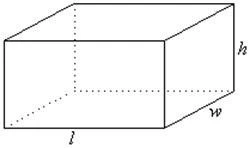The surface area is the total area of all the faces of a 3D shape.
The lateral surface area of a cuboid which has length, breadth, and height as (l, b, and h) can be explained as-
Lateral Surface Area = 2 (lh + wh) = 2h (l + w)

Topics and Subtopics of NCERT Class 9 Maths Chapter 13 – Surface Areas and Volumes

Chapter 13 Surface Areas and Volumes is divided further into topics and subtopics in which the content of previous knowledge of the student is given as well as the current syllabus is covered in the form of examples and exercises. Knowing the topics and subtopics of a given chapter helps the learners be aware of the whole content in a short form. This helps them to sort out each topic the way they want to while planning to study. Let us look at the Topics and Subtopics of NCERT Class 9 Maths Chapter 13 – surface areas and volumes, according to which the solutions are worked out on the website:

### Cube

Cardboard square box is an example of a cube. Therefore, we can say cube is a three-dimensional solid figure, which has 6 faces (sides), 12 edges and 8 vertices. In other words, it can also be described as –

A cuboid whose length, breadth and height are equal and if we consider the edge of the given cube as “a” then the surface area of the cube can be explained as –

2 (a x a + a x a + a x a)

= 2 (3a2)

i.e. 6a2

Therefore, the surface area of a cube is equal to 6a2

In the figure below cube with an edge “a” is shown –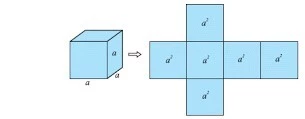When we measure only four sides of the cube and leave the upper and bottom surface then that area is known as lateral surface area, therefore it can be represented as follows for cube

The lateral surface area of a cuboid with side a is equal to 4a2

### Surface area of a Right Circular Cylinder

A cylinder which has a circle in its base portion and its elements are perpendicular (or we can say at the right angle) to its base is known as a right circular cylinder.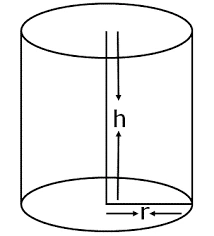In the figure above, a right circular cylinder is shown. For detecting the total surface area of a right circular cylinder, we need to know the following –

Curved surface area of a cylinder = area of rectangular portion i.e. length x breadth

= perimeter of the base of cylinder x h

= 2πr x h

Therefore, the curved surface area of a cylinder = 2πrh

Area of two circles in the cylinder of radius r is πr2

Therefore, the area of two circular bases is equal to 2πr2

Total surface area of right circular cylinder =2πrh + 2πr2

Hence, 2πr (r + h)

### Surface area of a Right Circular Cone

A cone whose axis is perpendicular to its base is called right circular cone. It can be seen that a cone is also made of a circle and a triangle.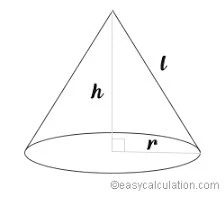Total surface area of a cone is equal to the curved surface area of the cone plus the area of the base- i.e πr2 + πlr, where ‘r’ is the radius of the base of the cone, and ‘l’ is the slant height of the cone.

Therefore, total surface area = πrl + πr2 = πr (l + r)

### Surface area of a Sphere

A geometrical figure, which is 3-dimensional, perfectly round, and circular just like a ball. It can also be defined as the set of all points that are at an equal distance from a single point in space. The distance from an outer point to the centre of the sphere is said as the radius(r) and the maximum straight distance from one side of the sphere to other side is called diameter (d).

height:249.8pt; width:374.05pt">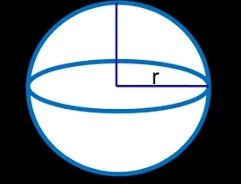Surface area of a sphere is equal to 4 times the area of circle of radius r

i.e. Surface area of a sphere = 4πr2

### Surface area of a Hemisphere

hemisphere is just half of a sphere, or we can define it as when we split a sphere into two parts   then it is called a hemisphere.

Therefore, the curved surface area of a hemisphere is equal to ½ of 4πr2

Hence, we get 2πr2

If we consider two faces of a hemisphere then the area is equal to 2πr2 + πr2

Hence – the total surface area of hemisphere = 3 πr2

### Volumes

As we know, solid objects occupy some space and when we measure this space it is called volume. Now we will learn about the volume of different solid objects.

Volume of cuboid – The measure of the space occupied in cuboid is called volume.

We can measure it as an area of the plane region occupied by each rectangle x height = volume

Volume of cuboid = length x breadth x height

In case of cube we take

V = edge x edge x edge

Therefore, the volume of cube = a3 (where a denotes edge of a cube)

### Volume of a cylinder

Just as cuboid

We can take the volume of cylinder = area of circular base x height

Therefore, volume of cylinder = πr2h

### Volume of a cone

We can say three times the volume of a cone is equal to the volume of a cylinder, which means the volume of a cone is one-third volume of a cylinder

Therefore - Volume of cone = 1/3 πr2h

### Volume of a Sphere

Volume of a sphere is equal to 4/3π times the cube of its radius.

Therefore, the volume of a sphere can be written as

= 4/3πr3

### Volume of a Hemisphere

We know hemisphere is half of a sphere, therefore, the volume of the hemisphere can be written as

½ of 4/3πr3

Hence, we get the volume of hemisphere = 2/3 πr3

## Significance of NCERT Mathematics Book Class 9

1. The NCERT book contains explanations of all the important concepts, which help students to understand the concepts in a comprehensive manner.
2. Different methods and concepts in this book are explained in an easy to understand language that makes it quite helpful for all the students.
3. Ample diagrams or figures and exercise on the same are illustrated in the NCERT book of the given chapter to make the mathematical concept easy-to-learn and understand.

The students are benefitted while practicing maths problems from the NCERT book and if they have any doubts, it can be cleared with the help of online video tutorials which are given on doubtnut.com, where the experts are available to help them in every possible way. On our website, all of these concepts are taught in video format that helps the students to learn the lessons with better concentration. All the doubts occurring during the learning process can be easily clarified by just posting the picture of the question on our website. Thus, Doubtnut is the right platform and students should download our play store app, doubtnut, for easy access to their queries. Our website makes the perfect tutorial platform for the students to face both board exams and the competitive exams in a stress-free way!